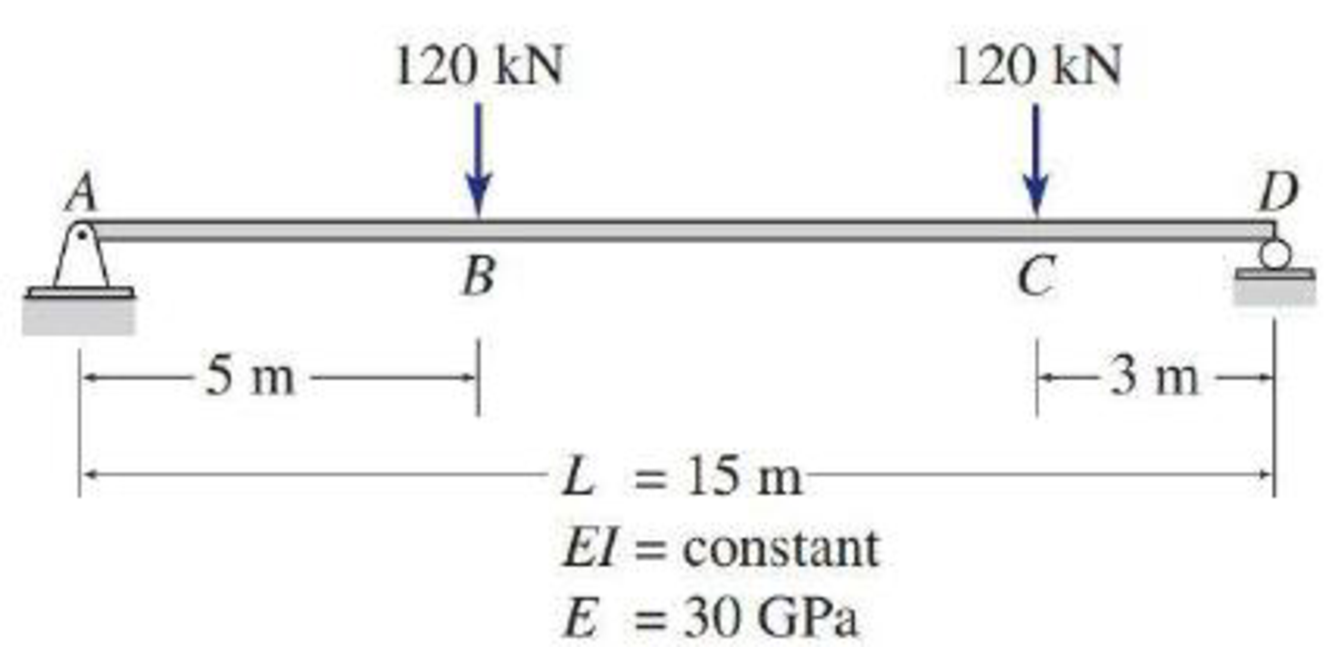# Using the conjugate-beam method, determine the smallest moments of inertia I required for the beams shown in Figs. P6.18 through P6.22, so that the maximum beam deflection does not exceed the limit of 1/360 of the span length (i.e., Δ max ≤ L / 360). FIG. P6.21, P6.47

#### Solutions

Chapter
Section
Chapter 6, Problem 47P
Textbook Problem
221 views

## Using the conjugate-beam method, determine the smallest moments of inertia I required for the beams shown in Figs. P6.18 through P6.22, so that the maximum beam deflection does not exceed the limit of 1/360 of the span length (i.e., Δmax ≤ L/ 360).FIG. P6.21, P6.47

To determine

Find the smallest moment of inertia (I) required for the beam if the deflection does not exceed the limit l/360 of the span length by using conjugate-beam method.

### Explanation of Solution

Given information:

The Young’s modulus is 30 GPa.

Calculation:

Consider the flexural rigidity EI of the beam is constant.

Show the free body diagram of the given beam as in Figure (1).

Refer Figure (1),

Consider upward forces are positive and downward forces are negative.

Consider clockwise moments are negative and counterclockwise moments are positive.

Determine the support reaction at A using the Equation of equilibrium;

MD=0RA×15(120×10)(120×3)=0RA=1,56015RA=104kN

Determine the reaction at support C;

V=0RA+RD120120=0RD=240104RD=136kN

Show the reactions of the given beam as in Figure (2).

Refer Figure (2),

Determine the bending moment at support A;

MA=(136×15)(120×12)(120×5)=2,0402,040=0

Determine the bending moment at B;

MB=(104×5)=520kNm

Determine the bending moment at C;

MC=(136×3)=408kNm

Determine the bending moment at D;

MD=+(104×15)(120×10)(120×3)=2,0402,040=0

Show the M/EI diagram of the given beam as in Figure (3).

Show the conjugate-beam as in Figure (4).

Determine the support reaction at A of a conjugate MEI diagram using the relation;

MD=0RA(15)+(12(520EI)(5)(13×5+10)(7)(408EI)(3+72)12(7)(520EI408EI)(23×7+3)12(3)(408EI)(23×3))=0RA=1EI(15,166.67+18,564+3,005.33+1,224)15RA=2,530.67kNm2EI

The maximum deflection will occur for the given beam at point M and take a distance of xM from point B.

Determine the intensity of load at xM using the relation;

wMxM=112kNm7mwM=1127xM

Determine the shear force at M using the relation;

SM=[RA+(12×b1×h1)+(xm×h2)12×wM×xM]=0

Here, b is the width and h is the height of respective triangle and rectangle.

Substitute 2,530.67EI for RA, 5 ft for b1, 520EI for h1, 520EI for h2, and 1127xM for wM.

[2,530.67EI+(12×5×520EI)+(xm×520EI)12×(1127xM)×xM]=01EI(2,530

### Still sussing out bartleby?

Check out a sample textbook solution.

See a sample solution

#### The Solution to Your Study Problems

Bartleby provides explanations to thousands of textbook problems written by our experts, many with advanced degrees!

Get Started

Find more solutions based on key concepts

Engineering Fundamentals: An Introduction to Engineering (MindTap Course List)

client (456) a. standard that outlines characteristics of how two devices communicate on a network b. guideline...

Enhanced Discovering Computers 2017 (Shelly Cashman Series) (MindTap Course List)

Give some examples of database connectivity options and what they are used for.

Database Systems: Design, Implementation, & Management

Which security protocols are used to protect e-mail?

Principles of Information Security (MindTap Course List)

Why is it important to minimize cable clutter in a rack?

Network+ Guide to Networks (MindTap Course List)

Briefly explain how HDFS and MapReduce are complementary to each other.

Database Systems: Design, Implementation, & Management

If your motherboard supports ECC DDR3 memory, can you substitute non-ECC DDR3 memory?

A+ Guide to Hardware (Standalone Book) (MindTap Course List)

Why is the torch tip raised as the cutting lever is depressed when cutting a hole?

Welding: Principles and Applications (MindTap Course List)